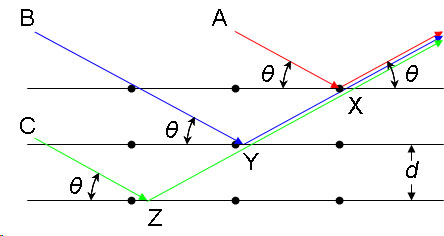Skip to main content

# Bragg's Law

The structures of crystals and molecules are often being identified using x-ray diffraction studies, which are explained by Bragg’s Law. The law explains the relationship between an x-ray light shooting into and its reflection off from crystal surface.

## Introduction

Bragg’s Law was introduced by Sir W.H. Bragg and his son Sir W.L. Bragg. The law states that when the x-ray is incident onto a crystal surface, its angle of incidence, $$\theta$$, will reflect back with a same angle of scattering, $$\theta$$. And, when the path difference, $$d$$ is equal to a whole number, $$n$$, of wavelength, a constructive interference will occur.

Consider a single crystal with aligned planes of lattice points separated by a distance d. Monochromatic X-rays A, B, and C are incident upon the crystal at an angle θ. They reflect off atoms X, Y, or Z.The path difference between the ray reflected at atom X and the ray reflected at atom Y can be seen to be 2YX. From the Law of Sines we can express this distance YX in terms of the lattice distance and the X-ray incident angle:

If the path difference is equal to an integer multiple of the wavelength, then X-rays A and B (and by extension C) will arrive at atom X in the same phase. In other words, given the following conditions:

then the scattered radiation will undergo constructive interference and thus the crystal will appear to have reflected the X-radiation. If, however, this condition is not satisfied, then destructive interference will occur.

## Bragg’s Law

$n\lambda = 2d\sin\theta$

where:

• $$\lambda$$ is the wavelength of the x-ray,
• $$d$$ is the spacing of the crystal layers (path difference),
• $$\theta$$ is the incident angle (the angle between incident ray and the scatter plane), and
• $$n$$ is an integer

The principle of Bragg’s law is applied in the construction of instruments such as Bragg spectrometer, which is often used to study the structure of crystals and molecules.

## References

1. Bragg, W.L. The Crystalline State: Volume I. New York: The Macmillan Company, 1934.
2. McQuarrie, Donald A. Physical Chemistry: A molecular Approach. Sausalito: University Science Books, 1997.
• Was this article helpful?